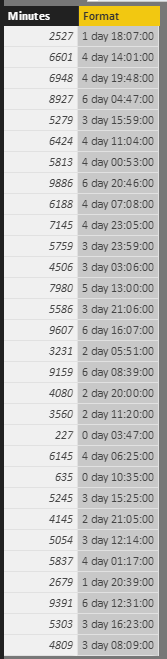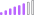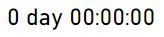cancel
Showing results for
Did you mean:Helper I

## Convert minutes into days and timeformat

Hi folks

I want to convert minutes into readable stuff for the endusers, e.g.:

I have 2.480,08 minutes

I want to format this in duration (but not in Time-Format) because the value is greater than 24 hours.

Result shoud be:

1 day 08:22:16

But without VAR - this slowdown extremly all my visualizations - isn´t it?

How to proceed?

1 ACCEPTED SOLUTIONHelper I

Hi - thanks, it works. Hopefully the VAR function works quick enough also with big data!

I have modified your DAX, to display the days or not, and hour work day has only 15 hours, so I divide through 900 instead of 1440.

WE Dauer gesamt =
var Tag=  INT(Lagerbeleg[WE Dauer in Min] / 900)
var Stunde=  INT(MOD(Lagerbeleg[WE Dauer in Min]; 900) / 60)
var Minute=  MOD(MOD(Lagerbeleg[WE Dauer in Min]; 900); 60)
var Sekunde= INT((Lagerbeleg[WE Dauer in Min] - INT(Lagerbeleg[WE Dauer in Min])) * 60)
return
IF(Tag = 0; "";
IF (Tag > 1; Tag & " Tage "; Tag & " Tag "))
& FORMAT(Stunde; "#00")
& ":"
& FORMAT(MINUTE; "#00")
&":"&
FORMAT(Sekunde; "#00")

13 REPLIES 13Regular Visitor

Hi I am looking to convert 10 Days 13 hours and 16 minutes to display in total hours 253.26 hours using DAX. Could someone help please?Community Support

Hi @JWE,

You can try to use belwo formula if it works on your side:

Format =
var dayNo=INT([Minutes]/1440)
var hourNo=INT(MOD([Minutes],1440)/60)
var minuteNO=MOD(MOD([Minutes],1440),60)
var secondNo=INT(([Minutes]-INT([Minutes]))*60)
return
dayNo&" day "&FORMAT(hourNo,"#00")&":"&FORMAT(minuteNO,"#00")&":"&FORMAT(secondNo,"#00")Regards,

Xiaoxin Sheng

Community Support Team _ Xiaoxin
If this post helps, please consider accept as solution to help other members find it more quickly.New Member

Hi! I will like know how to modify this formula so it can show seconds as wellFrequent Visitor

Hi @v-shex-msft  Thanks for this post. Could you pls help me in making the same query bit customised or precise . .

e.g.
1 day 1:00:00 hours
1 day 00:55:70 Minutes

that is if hour is present show postfix as hours
If time is less then hour show postfix as MinutesPost Patron

Hi @v-shex-msft, Could you tell me how this formula should be if I only want hours and minutes?

Format =
var dayNo=INT([Minutes]/1440)
var hourNo=INT(MOD([Minutes],1440)/60)
var minuteNO=MOD(MOD([Minutes],1440),60)
var secondNo=INT(([Minutes]-INT([Minutes]))*60)
return
dayNo&" day "&FORMAT(hourNo,"#00")&":"&FORMAT(minuteNO,"#00")&":"&FORMAT(secondNo,"#00")Anonymous
Not applicable

Hi @JWE,

The below mentioned DAX formula works for me as well. But when I tried to add two rows,I'm not getting the desired result because the value is taking as First or Last or Count but not Sum as mentioned below.

P.S: I'm using Impala as my Datasource.Thanks,

Akhil.Helper I

Hi Akhil

sorry but I have no idea. I only work with the DAX in the measure and display the results without using further field functions. In this case I get the correct sum.

Cheers JorgAnonymous
Not applicable

Hi @JWE,

Can I have the same formula work with the measure instead of creating a new column using the below DAX?

Time_Format =

var dayNo=INT([time]/1440)
var hourNo=INT(MOD([time],1440)/60)
var minuteNO=MOD(MOD([time],1440),60)
var secondNo=INT(([lingertime]-INT([time]))*60)
return
dayNo&" day "&FORMAT(hourNo,"#00")&":"&FORMAT(minuteNO,"#00")&":"&FORMAT(secondNo,"#00")

Thanks,

Akhil.Helper I

Yes, I think so, but you know measure is not the same like calculatd column.

Below measure I used:

WE Dauer gesamt =
var Tag=  INT(   Lagerbeleg[Hilfsmeasure WE Dauer in Min] / 900)
var Stunde=  INT(MOD(  Lagerbeleg[Hilfsmeasure WE Dauer in Min] ; 900) / 60)
var Minuten= INT(MOD(MOD( Lagerbeleg[Hilfsmeasure WE Dauer in Min] ; 900); 60))
var Sekunde= INT((   Lagerbeleg[Hilfsmeasure WE Dauer in Min]
- INT(   Lagerbeleg[Hilfsmeasure WE Dauer in Min] )) * 60)
return
IF(Tag = 0; "";
IF (Tag > 1; Tag & " Tage "; Tag & " Tag "))
& FORMAT(Stunde; "#00")
& ":"
& FORMAT(Minuten; "#00")

inside this I used:

Hilfsmeasure WE Dauer in Min =
SUMX(FILTER(
Lagerbeleg;
Lagerbeleg[Startdatum] = Lagerbeleg[Endedatum]
&& Lagerbeleg[Endezeit] = Lagerbeleg[MaxEndeZeit]
&& Lagerbeleg[LagerPosNr] = 1);
Lagerbeleg[Yakar Dauer Min gesamt])
+ (SUM(Lagerbeleg[Hilfsspalte WE > 1 T Sum]))
&":"&
FORMAT(Sekunde; "#00")Anonymous
Not applicable

Hi Jorg @JWE,

Sorry, I do not understand the below mentioned DAX. Can you please send me in plain English (US English)?

WE Dauer gesamt =
var Tag=  INT(   Lagerbeleg[Hilfsmeasure WE Dauer in Min] / 900)
var Stunde=  INT(MOD(  Lagerbeleg[Hilfsmeasure WE Dauer in Min] ; 900) / 60)
var Minuten= INT(MOD(MOD( Lagerbeleg[Hilfsmeasure WE Dauer in Min] ; 900); 60))
var Sekunde= INT((   Lagerbeleg[Hilfsmeasure WE Dauer in Min]
- INT(   Lagerbeleg[Hilfsmeasure WE Dauer in Min] )) * 60)
return
IF(Tag = 0; "";
IF (Tag > 1; Tag & " Tage "; Tag & " Tag "))
& FORMAT(Stunde; "#00")
& ":"
& FORMAT(Minuten; "#00")

inside this I used:

Hilfsmeasure WE Dauer in Min =
SUMX(FILTER(
Lagerbeleg;
Lagerbeleg[Startdatum] = Lagerbeleg[Endedatum]
&& Lagerbeleg[Endezeit] = Lagerbeleg[MaxEndeZeit]
&& Lagerbeleg[LagerPosNr] = 1);
Lagerbeleg[Yakar Dauer Min gesamt])
+ (SUM(Lagerbeleg[Hilfsspalte WE > 1 T Sum]))
&":"&
FORMAT(Sekunde; "#00")

I'm also thinking, can we convert the below DAX to SQL?

Time_Format =

var dayNo=INT([time]/1440)
var hourNo=INT(MOD([time],1440)/60)
var minuteNO=MOD(MOD([time],1440),60)
var secondNo=INT(([lingertime]-INT([time]))*60)
return
dayNo&" day "&FORMAT(hourNo,"#00")&":"&FORMAT(minuteNO,"#00")&":"&FORMAT(secondNo,"#00")

Thanks,

Akhil.Post Patron

Hello...Im Christián and I think I need something just like that...! my question is if  you are able to sum that column? eg: 4 days; 8:59+2 days:3:01 geting as a result= 6days:12:00?Helper I

Hello

yes, after I calculate the sum (like above measure, or something else) - and then I used a second measure to display days and also hours.

WE Dauer (Zeit) =
IF (
[Einl Dauer Sum] > 0;
FORMAT ( INT ( [Einl Dauer Sum] / ( 1 / 24 * 15 ) ); "#00T " )
& FORMAT ( MOD ( [Einl Dauer Sum]; ( 1 / 24 * 15 ) ); "HH:MM:SS" )
)

(In this case I use 15 - because our working days are not 24h only 15h.)Helper I

Hi - thanks, it works. Hopefully the VAR function works quick enough also with big data!

I have modified your DAX, to display the days or not, and hour work day has only 15 hours, so I divide through 900 instead of 1440.

WE Dauer gesamt =
var Tag=  INT(Lagerbeleg[WE Dauer in Min] / 900)
var Stunde=  INT(MOD(Lagerbeleg[WE Dauer in Min]; 900) / 60)
var Minute=  MOD(MOD(Lagerbeleg[WE Dauer in Min]; 900); 60)
var Sekunde= INT((Lagerbeleg[WE Dauer in Min] - INT(Lagerbeleg[WE Dauer in Min])) * 60)
return
IF(Tag = 0; "";
IF (Tag > 1; Tag & " Tage "; Tag & " Tag "))
& FORMAT(Stunde; "#00")
& ":"
& FORMAT(MINUTE; "#00")
&":"&
FORMAT(Sekunde; "#00")